# Streaming with flo_draw

I’m slowly starting to put together the final feature set of flo_draw 0.4. One of the new features is layer blending, which has its own new example. Implementing this required quite a bit of work to improve how `flo_draw` composites images, and it looks like this when demonstrating the multiplication blending mode (`cargo run --example mascot_shadow`):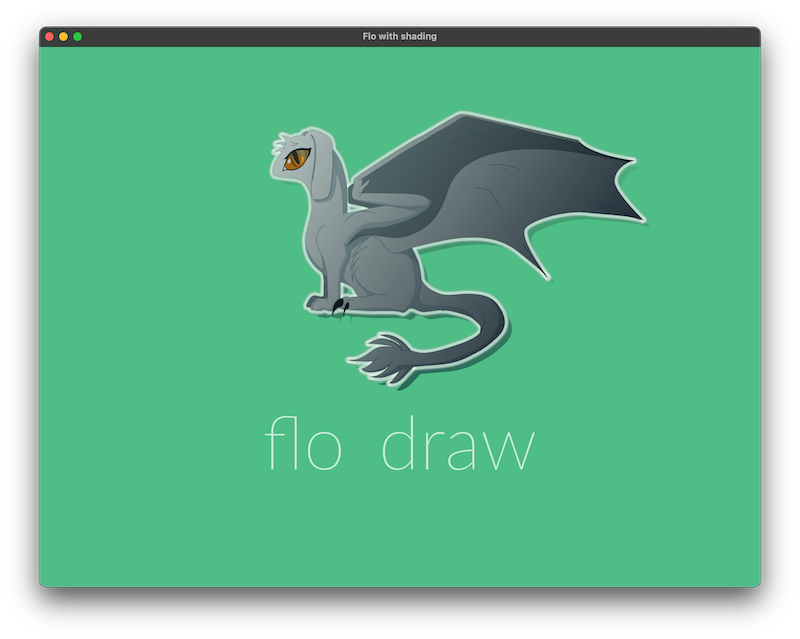This demo was created by decoding the instructions that make up the mascot image, adding them together using path arithmetic to make up a silhouette and finally drawing that silhouette on a layer underneath to make a drop shadow and again on top with a gradient to add a shading effect to the mascot as a whole. Finally the topmost layer is set to use the new multiply blending mode, which creates quite a satisfying effect for this kind of shading.

This nicely demonstrates quite a few of flo_draw's features, notably how v0.4 is much more capable when it comes to composing images. However, to do all of this, it needs to generate that silhouette vector to render underneath and on the top, and the way that happens is perhaps the most interesting part of this whole demo. Unlike the base image, which is converted from an SVG file, the silhouette needs to be entirely generated in code.

We want to generate the silouette and not just change the colours of the existing paths in order to be able to alpha-blend the shadow. That is, if we just render the list of paths with our alpha-blended gradient, the overlapping paths start to blend together and we get this: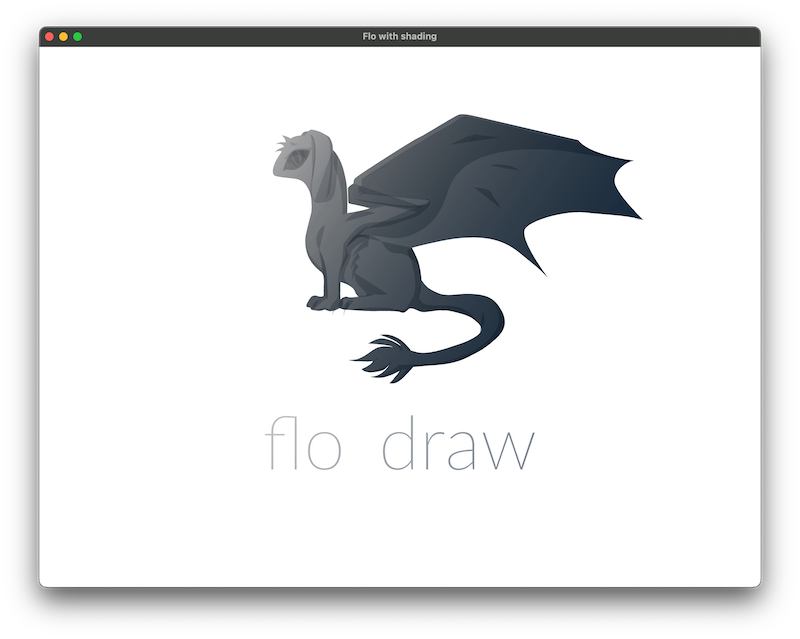Whereas if we generate a single path that's just the outline of the image, we get something that's much more useful for alpha blending: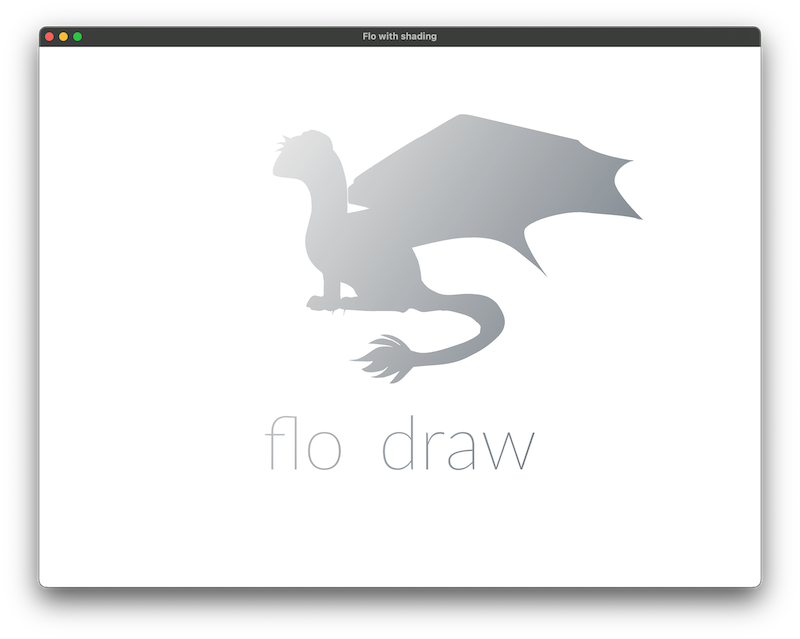Traditionally, this kind of effect is done by rendering the image to an off-screen buffer then processing it as a bitmap: replacing the colours but keeping the alpha value, for instance. The shadows that are underneath the windows in these screenshots are made by just such a technique. I'm working on building a framework for doing just such post-processing at the moment, but this particular demo is doing the same thing entirely by reprocessing the vector instructions that make up the image.

This is easy because `flo_draw` uses a streaming API rather than the more conventional method-calling approach, and that means we can intercept a rendering in flight and make changes to it. The code is very short for an effect that is quite tricky to pull off in other libraries. This API is actually separate from `flo_draw` itself - defined in the `flo_canvas` crate - making it possible to use it in other contexts where the rendering engine isn't required.

Here's how it works: we load the instructions to render the mascot into a `Vec`, and we use that to create a stream to pass in to `drawing_to_paths()`. This takes the rendering instructions and extracts just the paths that will be drawn.

``````let render_mascot   = stream::iter(mascot.clone().into_iter());
let mascot_paths    = drawing_to_paths::<SimpleBezierPath, _>(render_mascot);
let mascot_paths    = executor::block_on(async move { mascot_paths.collect::<Vec<_>>().await });``````

Now we need to add the paths together to create a single silouette path. Combining bezier paths is pretty tricky, but `flo_curves` has a simple API for performing just this operation:

``````let mut silouette = mascot_paths.clone();
for path in mascot_paths.iter().skip(1) {
silouette = path_add(&silouette, path, 0.1);
}``````

This is now ready to be rendered to the graphics context to create the shadow shape:

``````gc.new_path();
silouette.iter().for_each(|path| gc.bezier_path(path));
gc.fill();``````

We can go further, laying out text and converting it to a path, and distorting it in any way we like: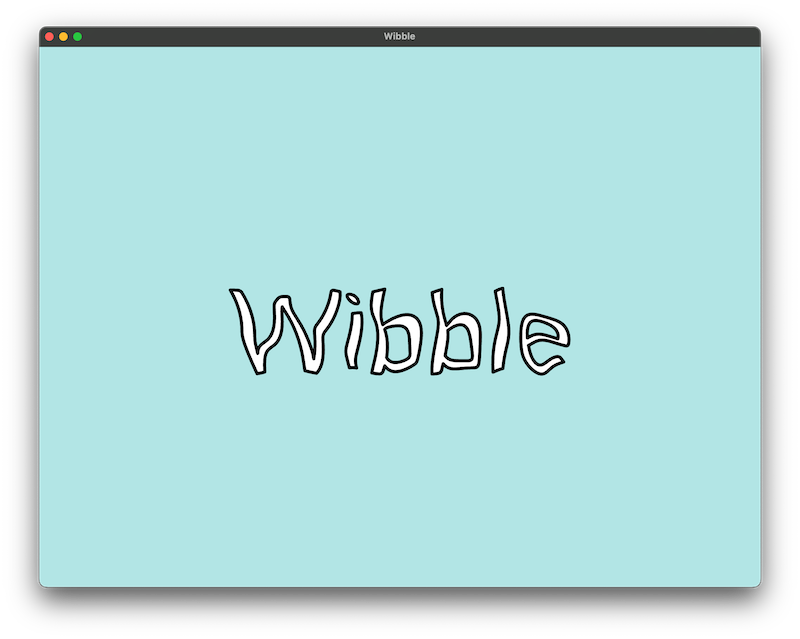The streaming design of the API means it's possible to mix and match layers of the rendering engine: it's just two method calls to take a stream that contains text rendering instructions and output a stream that contains everything represented as vector paths. The only reason it's two calls rather than one is that a rendering engine might want the text layout to draw with its own font renderer instead of drawing as vector paths.

``````// Describe the text rendering
let mut render_text = vec![];
render_text.define_font_data(FontId(1), Arc::clone(&lato));
render_text.set_font_size(FontId(1), 200.0);
render_text.draw_text(FontId(1), "Wibble".to_string(), x_pos as _, y_pos as _);

/// ... then convert to paths
let render_text = stream::iter(render_text.into_iter());
let text_paths  = drawing_with_laid_out_text(render_text);
let text_paths  = drawing_with_text_as_paths(text_paths);
let text_paths  = drawing_to_paths::<SimpleBezierPath, _>(text_paths);
let text_paths  = executor::block_on(async move { text_paths.collect::<Vec<_>>().await });``````

Generating the distorted path is just another call to `flo_curves` which has a convenient method for distorting any shape and generating the resulting bezier paths. This is an algorithm that moves all the points in circles, offset according to where they are in the image (which generates a sort of underwater rippling effect when animated)

``````let distorted_mascot = mascot_paths.par_iter()
.map(|(attributes, path_set)| (attributes, path_set.iter()
.map(move |path: &SimpleBezierPath| distort_path::<_, _, SimpleBezierPath>(path, |point: Coord2, _curve, _t| {
let distance    = point.magnitude();
let ripple      = (time_since_start / (f64::consts::PI * 500_000_000.0)) * 10.0;

let offset_x    = (distance / (f64::consts::PI*5.0) + ripple).sin() * amplitude * 0.5;
let offset_y    = (distance / (f64::consts::PI*4.0) + ripple).cos() * amplitude * 0.5;

Coord2(point.x() + offset_x, point.y() + offset_y)
}, 2.0, 1.0).unwrap())
.collect::<Vec<_>>()))
.collect::<Vec<_>>();``````

The renderer itself has a streaming approach, so it's possible to get to the instructions that would normally be sent to the GPU this way as well. The `flo_render_canvas` crate - with the help of `lyon` - deals with the job of turning the vector instructions described by `flo_canvas` into the sort of thing a GPU can understand (many buffers of triangles, essentially).

It's possible to obtain a stream of the instructions intended for the GPU using the `CanvasRenderer` structure with a stream of drawing instructions (and a bit of help from a pipe from the `desync` crate).

``````let renderer    = CanvasRenderer::new();
let renderer    = Arc::new(Desync::new(renderer));

let canvas_stream           = canvas_stream.ready_chunks(1000);
let mut gpu_instructions    = pipe(renderer, canvas_stream, |renderer, drawing_instructions| {
async move {
renderer.draw(drawing_instructions.into_iter())
.collect::<Vec<_>>()
.await
}.boxed()
}).map(|as_vectors| stream::iter(as_vectors)).flatten();``````

This can be drawn as 2D graphics again to visualise what the GPU is actually doing, then rendered again through the same rendering algorithm onto the screen in a sort of feedback loop.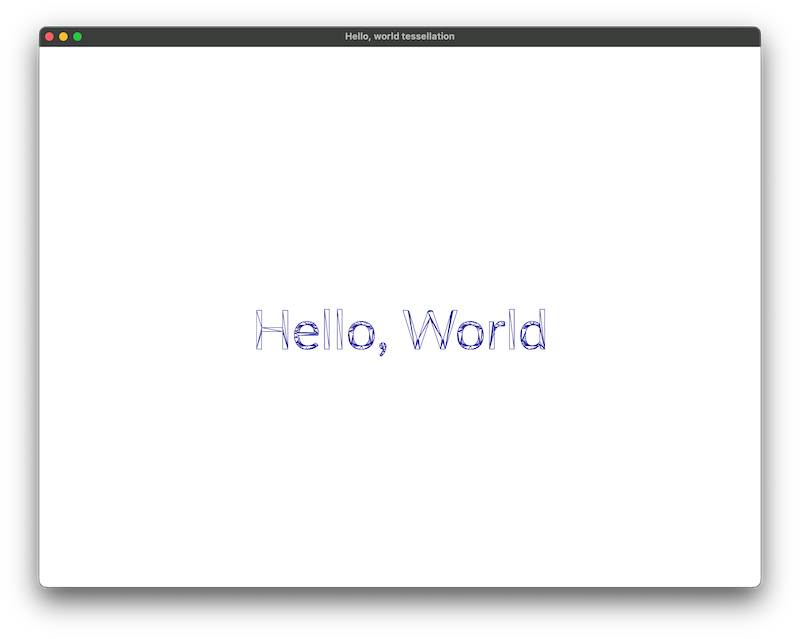Streaming APIs like this do have a downside: it's more expensive to send a message via a stream than it is to make a method call. This makes them unsuitable for inner loops but the inner loop here is really inside the GPU. They have a major upside over the more common 'method-based' APIs: it's very straightforward to intercept, reprocess and combine them into new forms. In `flo_draw`, this works as a force-multiplier: it's much faster to implement new functionality when everything just takes an input and an output and each component can be used independently of the whole.